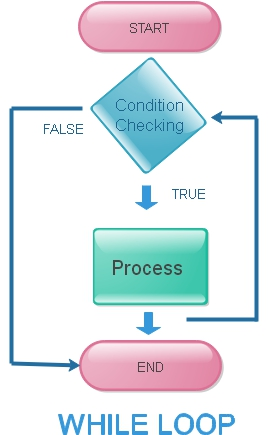# PHP while loop statement script and exampleWhile loop in PHP is very simple and similar to while loop in C programming. In PHP while loop the condition is checked at the beginning of the loop and the statement inside is executed till the end of the loop, then again the condition is checked and loop executed. Like this loop is continued till the condition became false and  the loop is then stopped.
``````while(condition checked){
code part to execute
}
``````
Here if the condition became false inside the loop then also the loop continue to execute till the next time the condition is checked.  Here is one simple example of while loop.
``````<?Php
\$j=5; // we assigned a value to the variable here
while(\$j <10 ){   // loop starts if condition is true
echo " value of \\$j= \$j <br>";
\$j=\$j+1;
}
?>``````
What is the output of this?
``````value of \$j= 5
value of \$j= 6
value of \$j= 7
value of \$j= 8
value of \$j= 9
``````
Here we are checking the while condition at the starting of the loop. So the execution of the loop will be done only if condition is satisfied at the beginning. We can read on the difference between do while loop and while loop here.

## Exit inside the loop by using break

Prematurely we can come out of the loop by using break statement. We used one if condition to check the value and then apply break statement.
``````<?Php
\$j=1; // we assigned a value to the variable here

while(\$j <10 ){   // loop starts if condition is true
echo " value of \\$j= \$j <br>";
if(\$j==7){
break;
}
\$j=\$j+1;
}
?>``````

## Display elements of an array by using While loop

Let us declare one array with some elements. Then by using while loop we can display the key and value of the array.
``````<?Php
\$a= array("Three", "two", "Four", "five","ten");
while(list(\$key,\$val) = each(\$a) ){
echo \$key . " > ". \$val . "<br>";
}
?>``````
The output is here
``````0 > Three
1 > two
2 > Four
3 > five
4 > ten``````

## Create ( 2 to 10 )multiplication table using While loop

To generate multiplication tables we will start with 2 table
Here is the simple code
``````\$i=2;
\$j=1;
while(\$j<= 10){ // Inner Loop
echo \$i*\$j." | ";
\$j=\$j+1;
}``````
This will display 2 table like this
``2 | 4 | 6 | 8 | 10 | 12 | 14 | 16 | 18 | 20 | ``
Now we will keep this loop inside another outer loop and change \$i value from 2 to 10. We will add a line break after each looping.
``````\$i=2;
\$j=1;
while(\$i<= 10){ // Outer Loop
while(\$j<= 10){ // Inner Loop
echo \$i*\$j." | ";
\$j=\$j+1;
} // End of Inner loop
echo "<br>";
\$j=1;
\$i=\$i+1;
}// End of outer loop``````
Output is here
``````2 | 4 | 6 | 8 | 10 | 12 | 14 | 16 | 18 | 20 |
3 | 6 | 9 | 12 | 15 | 18 | 21 | 24 | 27 | 30 |
4 | 8 | 12 | 16 | 20 | 24 | 28 | 32 | 36 | 40 |
5 | 10 | 15 | 20 | 25 | 30 | 35 | 40 | 45 | 50 |
6 | 12 | 18 | 24 | 30 | 36 | 42 | 48 | 54 | 60 |
7 | 14 | 21 | 28 | 35 | 42 | 49 | 56 | 63 | 70 |
8 | 16 | 24 | 32 | 40 | 48 | 56 | 64 | 72 | 80 |
9 | 18 | 27 | 36 | 45 | 54 | 63 | 72 | 81 | 90 |
10 | 20 | 30 | 40 | 50 | 60 | 70 | 80 | 90 | 100 | ``````
Visitors Rating